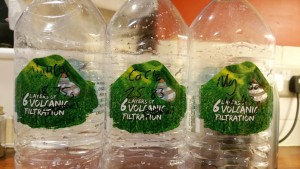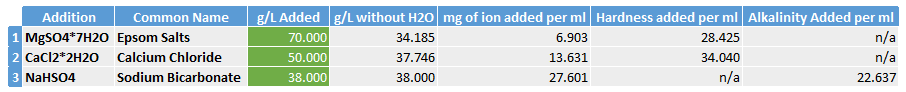So before we can begin to create water specifically for brewing coffee we need to create some stock solutions that we will use to add the required minerals into the brew water, I call these additions.

To create these additions I use chemicals that can easily be obtained combined with very low TDS water such as RO (Reverse Osmosis) or Distilled water. The additions are nothing more than very high concentration solutions created from each mineral that will allow us to easily make up brew water to a desired recipe.

I’d like to point out at this stage that you should always buy chemicals from reputable suppliers and ensure that they are as pure as possible. Lastly always read and adhere to the safety information supplied with them.

The chemicals I use are Magnesium Sulphate (Epsom Salts), Calcium Chloride and Sodium Bicarbonate. Epsom Salts are readily available from most chemists and Sodium Bicarbonate from any good supermarket as Bicarbonate of Soda. Calcium Chloride can be obtained from eBay or Amazon. I create a separate addition for each of these three chemicals so that I can add Magnesium, Calcium and Bicarbonate in varying quantities as required. You could simplify this down if you wanted but you will lose some flexibility.

To create the additions we need to understand what each chemical is made up of as well as what it will add to the brew water when we finally get round to making that. For my additions I’m adding the following

Addition 1 – 70.00g of Epsom Salts to 1L of RO water

Addition 2 – 50.00g of Calcium Chloride to 1L of RO water

Addition 3 – 38.00g of Sodium Bicarbonate to 1L of RO water

And here they are!The table below shows how how much hardness/alkalinity we will add to our brew water for each ml of each addition added.Ok so I said I wasn’t going to go into the calculations but I’ll try and explain for completeness sake.

The table above shows what happens when we add the addition compounds to 1L of very low TDS water. Taking Epsom Salts as an example, if we add 70.00g to 1L of RO water then almost 35.8g can be discounted as it’s just water (7H2O in the chemical formula), as we are adding this to water we just ignore it.

This leaves us with 34.19g of MgSO4.

Using the ratio of the molecular masses of Magnesium, Sulphur and Oxygen we can calculate that 6.903g of Magnesium Ion is added to the 1L of water. This means that if we took 1ml of this addition then it would contain 6.9mg of Magnesium ion to the new solution.

It’s important here to note that we need to maintain a good level of accuracy (2 or ideally 3 decimal places) in both our weights and calculations here as we are adding grams to solutions however we are looking to create brew water of only 20-50mg/L. If we weigh to zero decimal places then we could be out by up to 999mg in our additions which is no good! I weigh to 2 decimal places and find this adequate.

Carbonate chemistry uses the units of mg/L as Calcium Carbonate or Hardness. It took me a while to get my head around this but essentially what you are doing is converting the mass of your element or compound into the relative weight as if it were Calcium Carbonate. In our calculation we want to know how much hardness we are adding due to the Magnesium.

The atomic mass of Magnesium is 24.305

The molecular mass of Calcium Carbonate (CaCO3) is 100.0869

If we divide 100.0869 by 24.305 we get 4.12

This leads us to the statement that for every mg of Magnesium ion that we add to our brew water then the hardness added would be 4.12mg/L as CaCO3.

Therefore, if we add 1ml of our addition 1 to 1L of pure water then we are adding 6.9mg of Magnesium Ion. We then multiply this by 4.12 to get 28.43mg/L as CaCO3 hardness added.

The ratios used for the hardness conversion of Calcium is to multiply the mass of Ca+ ion by 2.497

We are also interested in calculating Alkalinity which is exactly the same process but instead of looking at the positive ion (Ca+/Mg+) we are looking at the negatively charged Bicarbonate ion, HCO3-

The conversion factor for Bicarbonate ion is to multiply mass of HCO3- by 0.82

I’ve already done this for you in the reference table above.

The Sodium (Na+), Sulphate (SO4-) and Chloride (Cl-) ions are not really relevant so can be ignored here.

I’ll try to get an additions calculator up here at some point but for now just make up the solutions as listed in the table above and we can move to Part 3 and starting making our own brew water.

• Really cool series. I’m looking forward to the rest.

•Brian
August 23, 2015 1:44 am

Have you experimented with potassium bicarbonate vs the sodium bicarbonate?

•Spencer Webb
August 23, 2015 2:38 pm

Hi Brian, I used potassium to try and keep the sodium levels to the scaa recommended 10ppm but it didn’t make any significant difference in taste.

•Ben Toovey
April 13, 2016 5:57 am

Hey Spencer, any chance of a solution recommendation for Magnesium Chloride? The maths melts my brain!

•Spencer Webb
April 15, 2016 2:10 am

Hi Ben, which Magnesium Chloride do you have? i.e. how many H2O’s does it have? The calcs will require this information. The problem with using it with the non-SS method is that you will start hitting levels of Chloride which can be bad for metals. Let me know and I’ll run up a simple calc for you.

•December 8, 2016 12:51 am

hi spencer, at the other article you prefer using calcium carbonate than sodium bicarbonate. If I change the sodium bicarbonate using calcium carbonate, can I use the bicarbonate formula? Or I must change the formula? thanks

•Spencer Webb
December 25, 2016 10:34 am

Hi there, the Calcium Carbonate method is different so you need to follow my guide, in my experiences simple calculations don’t seem to work for that method. If you are using the soda stream then you need to stick to sodium bicarbonate as calcium carbonate will not dissolve.

•Bhushan
January 27, 2017 8:21 pm

Hello, Great article. I have a question. The chemical formula for Sodium Bicarbonate is NaHCO3. How are you getting NaHSO4 which is Sodium Sulphate. Am I missing something here?

•Spencer Webb
February 16, 2017 5:27 pm

Sorry it’s a typo, should be Sodium Bicarb 🙂

•kurt
September 24, 2017 8:28 pm

thx ben. interested to know what brand / model of scale do use to get the decimal accuracy you recommend. thx.

• Spencer! Any chance you could calculate your formula to mirror the simplicity of the new Third Wave Water Additive?
Doing so would find me making a 1 cup mason jar filled with the three chemical elements in the proportions you determine.
I would then simply add the correct amount to a 1-gallon container of RO water and continue to use it up and refill accordingly.

Simplfy, simplify, simplivy. 🙂

Jerry

•